# Electronic Science - Online Test

Q1. Circuit turn-off time of an SCR is defined as the time
Explaination / Solution:
No Explaination.

Q2. The output Y of the logic circuit given below isExplaination / Solution: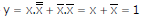Q3.
The bridge circuit shown in the figure below is used for the measurement of an unknown element ZX. The bridge circuit is best suited when ZX is a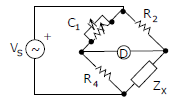Explaination / Solution:

Q4. Work done by a uniform electric field E in moving a charge q a distance d from a to b is
Explaination / Solution:

Force and the work done
Q5. The r.m.s value of the current i(t) in the circuit shown below is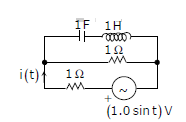Explaination / Solution: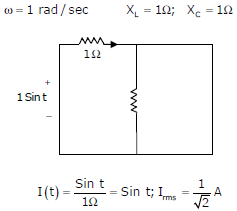Q6. What is the minimum number of gates required to implement the Boolean function (AB + C) if we have to use only 2-input NOR gates?
Explaination / Solution:
No Explaination.

Q7.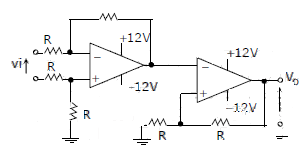The CORRECT transfer characteristic is

Explaination / Solution:

It is a Schmitt trigger and phase shift is zero.

Q8. A wire is perpendicular to the plane of the paper. A ring of compass needles surrounds the wire in the plane of the paper with center of ring being the center of the wire .Initially there is no current in the wire. What happens after a steady dc current is established in the wire?
Explaination / Solution:

The current carrying wire has a magnetic field around it and the lines of force are in the form of concentric circles with their centers on the wire.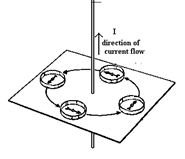Q9. Two cells of 1.25 V and 0.75 V are connected in series with anode of one connected to cathode of the other . The effective voltage will be
Explaination / Solution:

The cells support each other.
Q10. which of the following statements about earth's magnetism is correct
Explaination / Solution:
No Explaination.